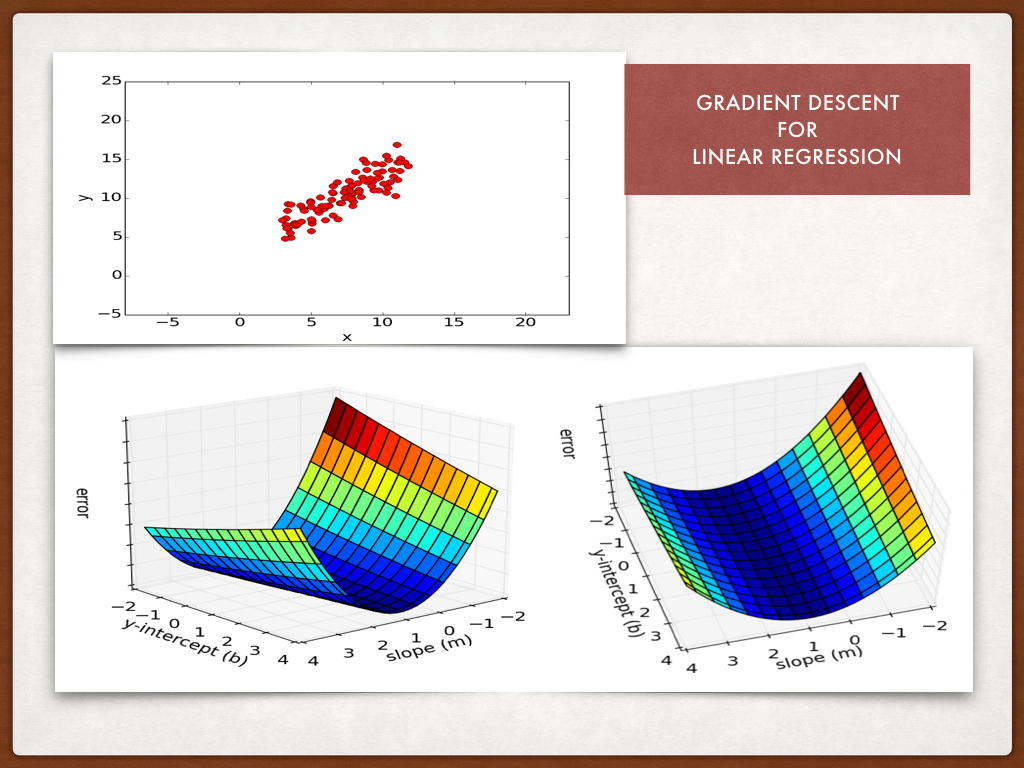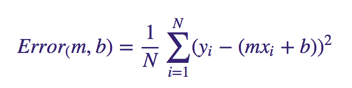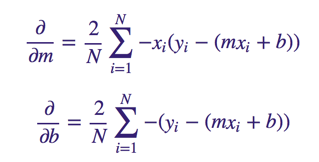Over a million developers have joined DZone.{{announcement.body}}
{{announcement.title}}

# A Program for Linear Regression With Gradient Descent

DZone 's Guide to

# A Program for Linear Regression With Gradient Descent

### Ruby isn't known as a primary language for math. But its functional syntax for operating on collections and ability to handle formatted files cleanly make it an elegant choice to understand what an algorithm is doing.

· Big Data Zone ·
Free Resource

Comment (0)

Save
{{ articles.views | formatCount}} Views

I took a Python program that applies gradient descent to linear regression and converted it to Ruby. But first, a recap: we use linear regression to do numeric prediction.

If x is an input (independent) variable and y is an output (dependent) variable, we come up with an initial formula (equation) that shows the mathematical relation between them.

Next we take each value of x and calculate the value of y using our initial equation. The difference between the calculated value of y and the actual y value corresponding to the x value is the error. The error values are summed up by which we arrive at another equation. We minimize this equation using the gradient descent algorithm so that we come up with the best-fit equation.When I was searching the web on this topic, I came across this page “An Introduction to Gradient Descent and Linear Regression” by Matt Nedrich in which he presents a Python example. The program finds the best-fit line for a given data set of x and y values. Good catch for me. For practice, I took Matt’s program and re-wrote it in Ruby.

I liked Matt’s blog article, so I am giving parts of it below but with my Ruby snippets.

To compute the error for a given line, we’ll iterate through each (x, y) point in our data set and sum the square distances between each point’s y value and the candidate line’s y value (computed at mx + b).

Formally, this error function will look like:``````0.upto points.length-1 do |i|
x = points[i]
y = points[i]
totalError += (y - (m * x + b)) ** 2
end

When we run a gradient descent search, we will start from some location on this surface and move downhill to find the line with the lowest error. To run gradient descent on this error function, we first need to compute its gradient. The gradient will act like a compass and always point us downhill.

To compute it, we will need to differentiate our error function. Since our function is defined by two parameters (m and b), we will need to compute a partial derivative for each. The derivatives work out to be:``````0.upto points.length-1 do |i|
x = points[i]
y = points[i]
m_gradient += -(2/n) * x * (y - ((m_current * x) + b_current))
b_gradient += -(2/n) * (y - ((m_current * x) + b_current))
end
new_m = m_current - (learningRate * m_gradient)
new_b = b_current - (learningRate * b_gradient)``````

Finally, we come to the complete program:

``````require 'csv'

# y = mx + b
# m is slope, b is y-intercept
def compute_error_for_line_given_points(b, m, points)
totalError = 0
0.upto points.length-1 do |i|
x = points[i]
y = points[i]
totalError += (y - (m * x + b)) ** 2
end
end

n = points.length + 0.0

0.upto points.length-1 do |i|
x = points[i]
y = points[i]
m_gradient += -(2/n) * x * (y - ((m_current * x) + b_current))
b_gradient += -(2/n) * (y - ((m_current * x) + b_current))
end
new_m = m_current - (learningRate * m_gradient)
new_b = b_current - (learningRate * b_gradient)
return [new_b, new_m]
end

def gradient_descent_runner(points, starting_b, starting_m, learning_rate, num_iterations)
b = starting_b
m = starting_m

0.upto num_iterations-1 do |i|
b, m = step_gradient(b, m, points, learning_rate)
end
return [b, m]
end

def run()
learning_rate = 0.0001
initial_b = 0 # initial y-intercept guess
initial_m = 0 # initial slope guess
num_iterations = 1000
puts "Starting gradient descent at b = #{initial_b}, m = #{initial_m}, error = #{compute_error_for_line_given_points(initial_b, initial_m, points)}"
puts "Running..."
(b, m) = gradient_descent_runner(points, initial_b, initial_m, learning_rate, num_iterations)
puts "After #{num_iterations} iterations b = #{b}, m = #{m}, error = #{compute_error_for_line_given_points(b, m, points)}"
end

run()``````

The file "data.cv" required to run this program is available at this link.

Topics:
math ,linear regression ,ruby

Comment (0)

Save
{{ articles.views | formatCount}} Views

Published at DZone with permission of

Opinions expressed by DZone contributors are their own.